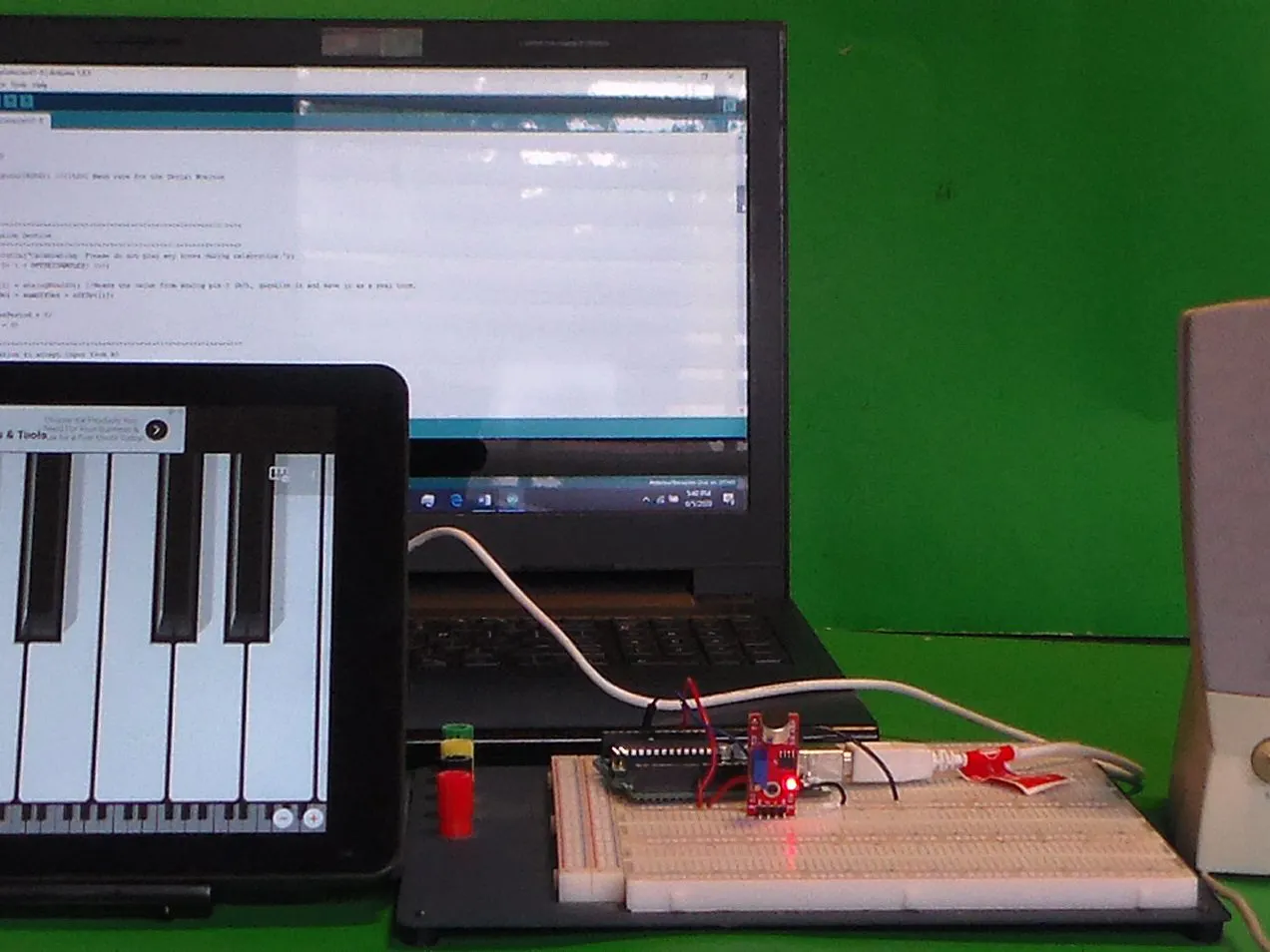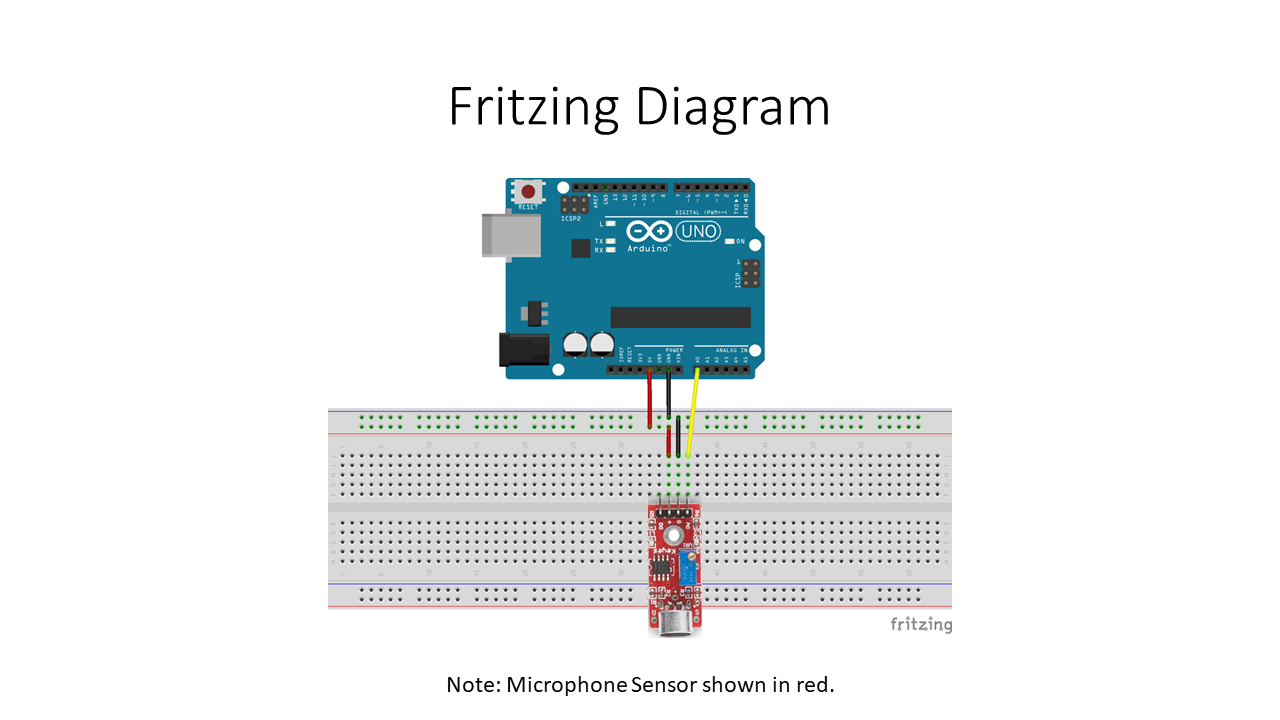Project tutorial# Musical Note Detector © GPL3+

This code/sketch displays the approximate frequency as well as the musical note played on an electronic keyboard or piano app.

• 912 views
• 1 respect

## Apps and online services

 Mini Piano LT

This code/sketch displays the approximate frequency as well as the musical note played on an electronic keyboard or piano app. For this project, the analog output from the

sound module detector is sent to the A0 analog input of the Arduino Uno. The analog signal is sampled and quantized (digitized). Autocorrelation, weighting and tuning code is used to

find fundamental frequency using the first 3 periods. The approximate fundamental frequency is then compared to frequencies in octaves 3, 4, and 5 range to determine the closest musical

note frequency. Finally the guessed note for the closest frequency is printed to the screen.

## Code

##### Musical Note DetectorC/C++
```/*
File/Sketch Name: MusicalNoteDetector

Version No.: v1.0 Created 7 June, 2020

Original Author: Clyde A. Lettsome, PhD, PE, MEM

Description:  This code/sketch displays the approximate frequency as well as the musical note played on an electronic keyboard or piano app. For this project, the analog output from the
sound module detector is sent to the A0 analog input of the Arduino Uno. The analog signal is sampled and quantized (digitized). Autocorrelation, weighting and tuning code is used to
find fundamental frequency using the first 3 periods. The approximate fundamental frequency is then compared to frequencies in octaves 3, 4, and 5 range to determine the closest musical
note frequency. Finally the guessed note for the closest frequency is printed to the screen.

License: This program is free software; you can redistribute it and/or modify it under the terms of the GNU General Public License (GPL) version 3, or any later

Notes: Copyright (c) 2020 by C. A. Lettsome Services, LLC

*/
#define SAMPLES 128             //Max 128 for Arduino Uno.
#define SAMPLING_FREQUENCY 2048 //Fs = Based on Nyquist, must be 2 times the highest expected frequency.
#define OFFSETSAMPLES 40  //used for calabrating purposes
#define TUNER -3    //Adjust until C3 is 130.50

float samplingPeriod;
unsigned long microSeconds;

int X[SAMPLES]; //create vector of size SAMPLES to hold real values
float autoCorr[SAMPLES]; //create vector of size SAMPLES to hold imaginary values
float storedNoteFreq = {130.81, 138.59, 146.83, 155.56, 164.81, 174.61, 185, 196, 207.65, 220, 233.08, 246.94};

int sumOffSet = 0;
int offSet[OFFSETSAMPLES]; //create offset vector
int avgOffSet; //create offset vector

int i, k, periodEnd, periodBegin, period, adjuster, noteLocation, octaveRange;
float  maxValue, minValue;
long sum;
int thresh = 0;
int numOfCycles = 0;
float signalFrequency, signalFrequency2, signalFrequency3, signalFrequencyGuess, total;
byte state_machine = 0;
int samplesPerPeriod = 0;

void setup()
{
Serial.begin(115200); //115200 Baud rate for the Serial Monitor
}

void loop()
{
//*****************************************************************
//Calabration Section
//*****************************************************************
Serial.println("Calabrating. Please do not play any notes during calabration.");
for (i = 0; i < OFFSETSAMPLES; i++)
{
offSet[i] = analogRead(0); //Reads the value from analog pin 0 (A0), quantize it and save it as a real term.
//Serial.println(offSet[i]); //use this to adjust the sound detection module to approximately half or 512 when no sound is played.
sumOffSet = sumOffSet + offSet[i];
}
samplesPerPeriod = 0;
maxValue = 0;

//*****************************************************************
//Prepare to accept input from A0
//*****************************************************************
avgOffSet = round(sumOffSet / OFFSETSAMPLES);
Serial.println("Counting down.");
delay(1000);  //pause for 1 seconds
Serial.println("3");
delay(1000);  //pause for 1 seconds
Serial.println("2");
delay(1000);  //pause for 1
Serial.println("1");
delay(1000);  //pause for 1 seconds
delay(250);  //pause for 1/4 second for reaction time

//*****************************************************************
//Collect SAMPLES samples from A0 with sample period of samplingPeriod
//*****************************************************************
samplingPeriod = 1.0 / SAMPLING_FREQUENCY; //Period in microseconds
for (i = 0; i < SAMPLES; i++)
{
microSeconds = micros();    //Returns the number of microseconds since the Arduino board began running the current script.
X[i] = analogRead(0); //Reads the value from analog pin 0 (A0), quantize it and save it as a real term.

/*remaining wait time between samples if necessary in seconds */
while (micros() < (microSeconds + (samplingPeriod * 1000000)))
{
//do nothing just wait
}
}

//*****************************************************************
//Autocorrelation Function
//*****************************************************************

for (i = 0; i < SAMPLES; i++) //i=delay
{
sum = 0;
for (k = 0; k < SAMPLES - i; k++) //Match signal with delayed signal
{
sum = sum + (((X[k]) - avgOffSet) * ((X[k + i]) - avgOffSet)); //X[k] is the signal and X[k+i] is the delayed version
}
autoCorr[i] = sum / SAMPLES;

// First Peak Detect State Machine
if (state_machine==0 && i == 0)
{
thresh = autoCorr[i] * 0.5;
state_machine = 1;
}
else if (state_machine == 1 && i>0 && thresh < autoCorr[i] && (autoCorr[i]-autoCorr[i-1])>0) //state_machine=1, find 1 period for using first cycle
{
maxValue = autoCorr[i];

}
else if (state_machine == 1&& i>0 && thresh < autoCorr[i-1] && maxValue == autoCorr[i-1] && (autoCorr[i]-autoCorr[i-1])<=0)
{
periodBegin = i-1;
state_machine = 2;
numOfCycles = 1;
samplesPerPeriod = (periodBegin - 0);
period = samplesPerPeriod;
adjuster = TUNER+(50.04 * exp(-0.102 * samplesPerPeriod));
signalFrequency = ((SAMPLING_FREQUENCY) / (samplesPerPeriod))-adjuster; // f = fs/N
}
else if (state_machine == 2 && i>0 && thresh < autoCorr[i] && (autoCorr[i]-autoCorr[i-1])>0) //state_machine=2, find 2 periods for 1st and 2nd cycle
{
maxValue = autoCorr[i];
}
else if (state_machine == 2&& i>0 && thresh < autoCorr[i-1] && maxValue == autoCorr[i-1] && (autoCorr[i]-autoCorr[i-1])<=0)
{
periodEnd = i-1;
state_machine = 3;
numOfCycles = 2;
samplesPerPeriod = (periodEnd - 0);
signalFrequency2 = ((numOfCycles*SAMPLING_FREQUENCY) / (samplesPerPeriod))-adjuster; // f = (2*fs)/(2*N)
maxValue = 0;
}
else if (state_machine == 3 && i>0 && thresh < autoCorr[i] && (autoCorr[i]-autoCorr[i-1])>0) //state_machine=3, find 3 periods for 1st, 2nd and 3rd cycle
{
maxValue = autoCorr[i];
}
else if (state_machine == 3&& i>0 && thresh < autoCorr[i-1] && maxValue == autoCorr[i-1] && (autoCorr[i]-autoCorr[i-1])<=0)
{
periodEnd = i-1;
state_machine = 4;
numOfCycles = 3;
samplesPerPeriod = (periodEnd - 0);
signalFrequency3 = ((numOfCycles*SAMPLING_FREQUENCY) / (samplesPerPeriod))-adjuster; // f = (3*fs)/(3*N)
}
}

//*****************************************************************
//Result Analysis
//*****************************************************************
if (samplesPerPeriod == 0)
{
Serial.println("Hmm..... I am not sure. Are you trying to trick me?");
}
else
{
//prepare the weighting function
total = 0;
if (signalFrequency !=0)
{
total = 1;
}
if(signalFrequency2 !=0)
{
total = total + 2;
}
if (signalFrequency3 !=0)
{
total = total + 3;
}

//calculate the frequency using the weighting function
signalFrequencyGuess = ((1/total) * signalFrequency) + ((2/total) * signalFrequency2) + ((3/total) * signalFrequency3); //find a weighted frequency
Serial.print("The note you played is approximately ");
Serial.print(signalFrequencyGuess);     //Print the frequency guess.
Serial.println(" Hz.");

//find octave range based on the guess
octaveRange=3;
while (!(signalFrequencyGuess >= storedNoteFreq-7 && signalFrequencyGuess <= storedNoteFreq+7 ))
{
for(i = 0; i < 12; i++)
{
storedNoteFreq[i] = 2 * storedNoteFreq[i];
}
octaveRange++;
}

//Find the closest note
minValue = 10000000;
noteLocation = 0;
for (i = 0; i < 12; i++)
{
if(minValue> abs(signalFrequencyGuess-storedNoteFreq[i]))
{
minValue = abs(signalFrequencyGuess-storedNoteFreq[i]);
noteLocation = i;
}
}

//Print the note
Serial.print("I think you played ");
if(noteLocation==0)
{
Serial.print("C");
}
else if(noteLocation==1)
{
Serial.print("C#");
}
else if(noteLocation==2)
{
Serial.print("D");
}
else if(noteLocation==3)
{
Serial.print("D#");
}
else if(noteLocation==4)
{
Serial.print("E");
}
else if(noteLocation==5)
{
Serial.print("F");
}
else if(noteLocation==6)
{
Serial.print("F#");
}
else if(noteLocation==7)
{
Serial.print("G");
}
else if(noteLocation==8)
{
Serial.print("G#");
}
else if(noteLocation==9)
{
Serial.print("A");
}
else if(noteLocation==10)
{
Serial.print("A#");
}
else if(noteLocation==11)
{
Serial.print("B");
}
Serial.println(octaveRange);
}
//*****************************************************************
//Stop here. Hit reset button on Arduino to restart
//*****************************************************************
while (1);
}
```

## Schematics#### Author• 5 projects
• 13 followers

#### Published on

June 8, 2020
See similar projects
you might like

#### Audio Frequency Detector

Project tutorial by calettso

• 5,811 views
• 1 comment
• 10 respects

#### Make Musical Instrument Using Arduino and Flick Large

Project tutorial by LanmiLab

• 4,019 views
• 16 respects

#### Mechanical Musical Arm

Project showcase by PIYUSH_K_SINGH

• 1,232 views
• 1 respect

#### Arduino Musical Tesla Coil

Project in progress by Brandon Michelsen

• 13,033 views
• 17 respects

#### Motion-Activated Musical Trophy

Project tutorial by Alex Wulff

• 1,986 views